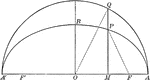### Corresponding Points in an Ellipse and Circle

Illustration of half of an ellipse. "The ordinates of two corresponding points in an ellipse and its…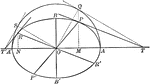### Conjugate Diameters of an Ellipse

Illustration showing that if one diameter is conjugate to a second, the second is conjugate to the first.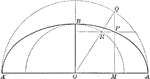### Construction of an Ellipse

Illustration of half of an ellipse and its auxiliary circle used to construct an ellipse by points,…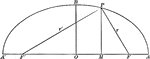### Focal Radii of an Ellipse

Illustration of half of an ellipse. "If d denotes the abscissa of a point of an ellipse, r and r' its…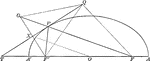### Line Bisecting Angle Between Focal Radii on Ellipse

Illustration of half of an ellipse. "If through a point P of an ellipse a line is drawn bisecting the…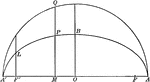### Ordinate and Major Axis of Ellipse

Illustration of half of an ellipse. The square of the ordinate of a point in an ellipse is to the product…### Parallel Tangents to an Ellipse

Illustration showing that tangents drawn at the ends of any diameter are parallel to each other.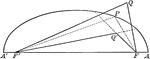### Point Distances to Foci on Ellipse

Illustration of half of an ellipse. "The sum of the distances of any point from the foci of an ellipse…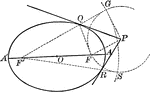### Tangent From External Point to an Ellipse

Illustration of how to draw a tangent to an ellipse from an external point.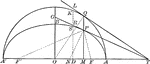### Tangents to an Ellipse

Illustration showing the tangents drawn at two corresponding points of an ellipse and its auxiliary…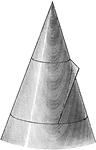### Conic Section Showing Parabola

Illustration showing the definition of a parabola as a conic section. "The section of a right circular…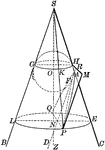### Conic Section Showing Parabola

Illustration showing the definition of a parabola as a conic section. "The section of a right circular…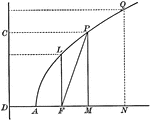### Parabola With Coordinates and Latus Rectum

Illustration of a parabola showing that any point of a parabola is the mean proportional between the…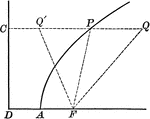### Point on Parabola

Illustration of a parabola showing point P is equidistant to the focus and the directrix.### Point on Parabola

Illustration of a parabola. "If a line PT is drawn from any point P of the curve, bisecting the angle…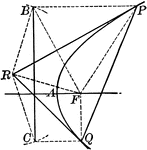### Tangent to a Parabola

Illustration of a tangent line drawn from an external point to a parabola.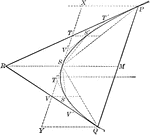### Tangents and Chords of a Parabola

Illustration showing that the area of a parabolic segment made by a chord is two thirds the area of…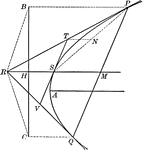### Tangents to a Parabola

Illustration showing that if two tangents RP and RQ are drawn from a point R to a parabola, the line…